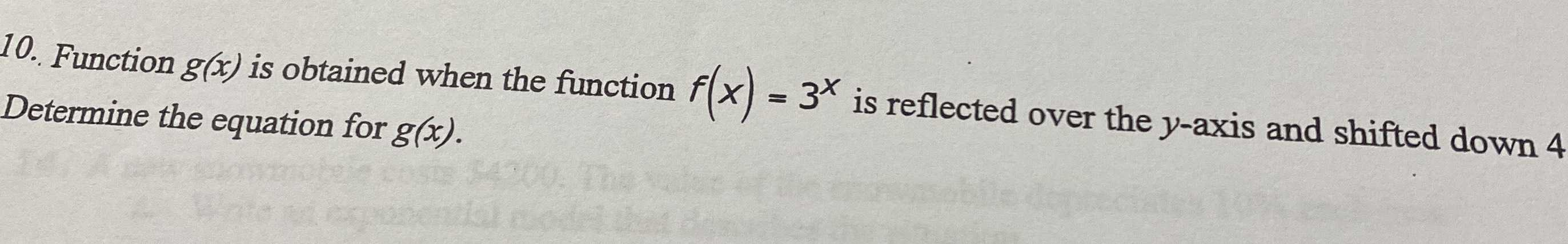### ¿Todavía tienes preguntas de matemáticas?

Pregunte a nuestros tutores expertos
Algebra
Pregunta10. Function $$g ( x )$$ is obtained when the function $$f ( x ) = 3 ^ { x }$$ is reflected over the $$y$$ -axis and shifted down $$4$$ Determine the equation for $$g ( x )$$ .

g(x) = 3$$^{- x}$$ - 4